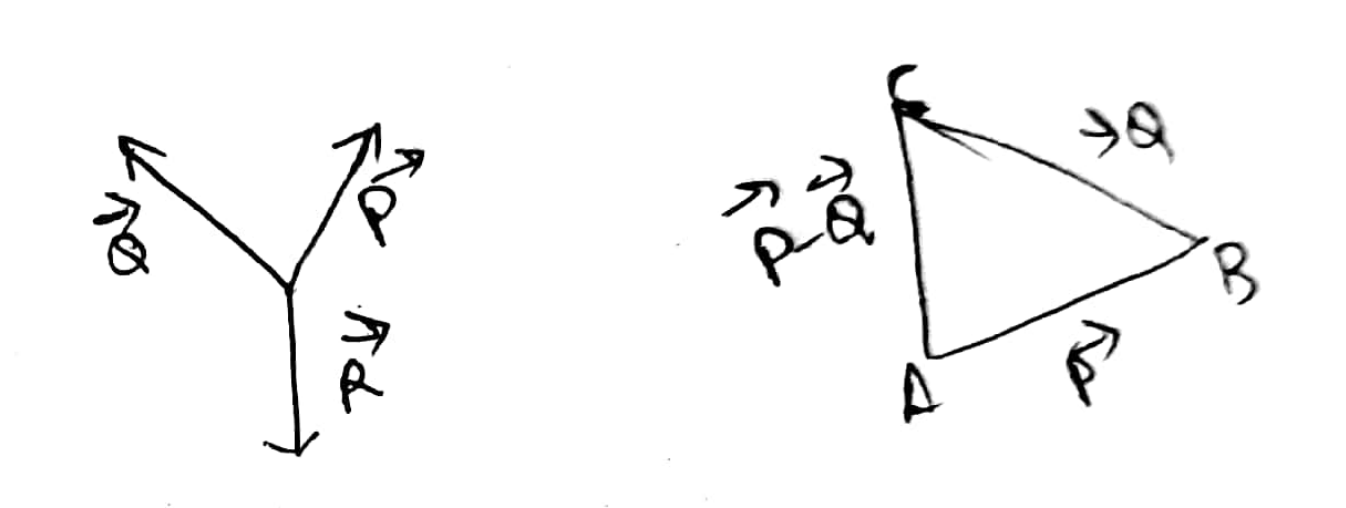# Physics - Triangle Laws of Vectors

Triangle Laws of Vectors

Statement :

" If two vectors are represented in magnitude and direction by the two sides of a triangle taken in order, then the third side of the triangle taken in reverse order represents their resultant in magnitude and direction "Explanation :

Suppose two vectors P and Q are acting at the point Q simultaneously. P and Q are represented in both magnitude and direction by AB and BC respectively. Then the third side taken in the reverse order i.e AC represent their resultant in both magnitue and direction.

P + Q = -R

P + Q + R = 0

which means that the three vectors P , Q and R keep the pont 'O' in equilibrium.

Post By : Ashish Maurya 26 May, 2020 421 views Physics